#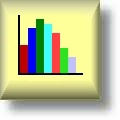Graph Worksheets

## Learning to Work with Charts and Graphs

Here is a graphic preview for all of the graph worksheets. You can select different variables to customize these graphing worksheets for your needs. The graph worksheets are randomly created and will never repeat so you have an endless supply of quality graph worksheets to use in the classroom or at home. We also produce blank Standard Graphing paper, Coordinate Plane Graphing Paper, and Polar Coordinate Graphing Paper for your use. Our graphing worksheets are free to download, easy to use, and very flexible.

## Quick Link for All Graph Worksheets

Click the image to be taken to that Graph Worksheet.

##### Single Line GraphingGraph Worksheets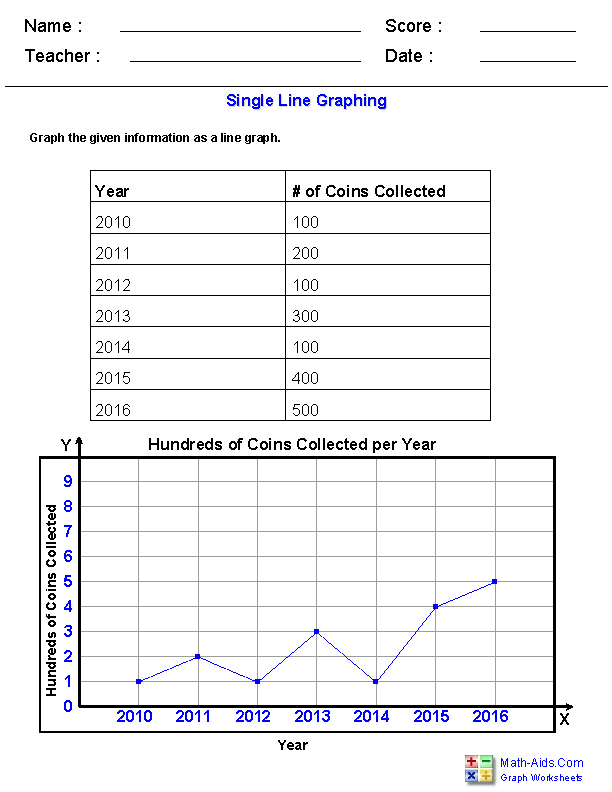##### Single Line GraphComprehensionGraph Worksheets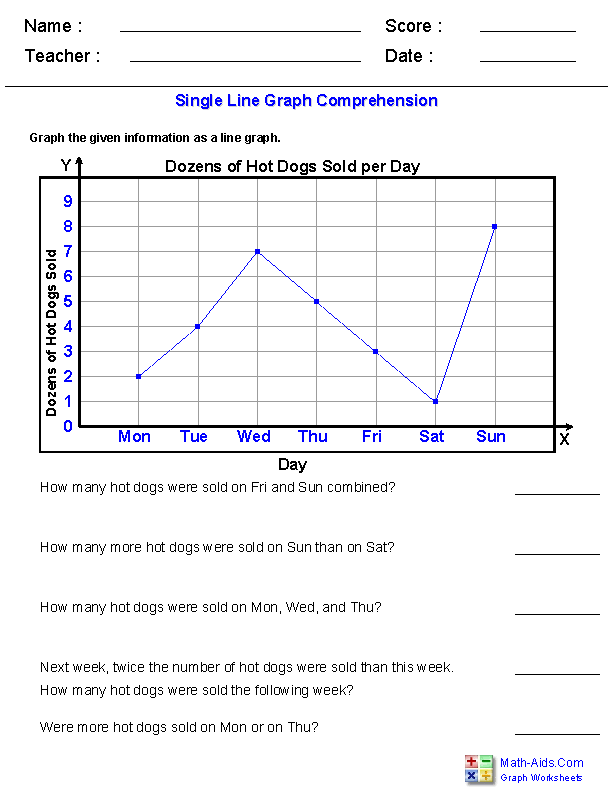##### Double Line GraphingGraph Worksheets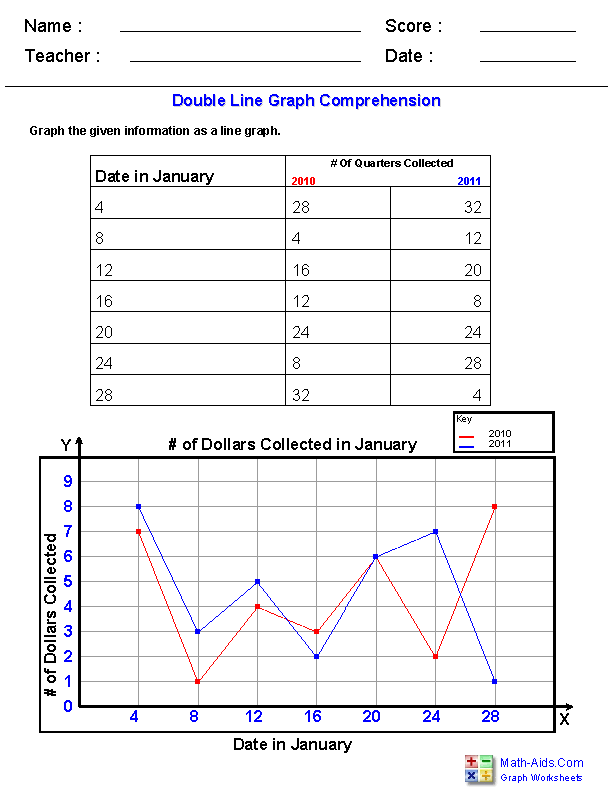##### Double Line GraphComprehensionGraph Worksheets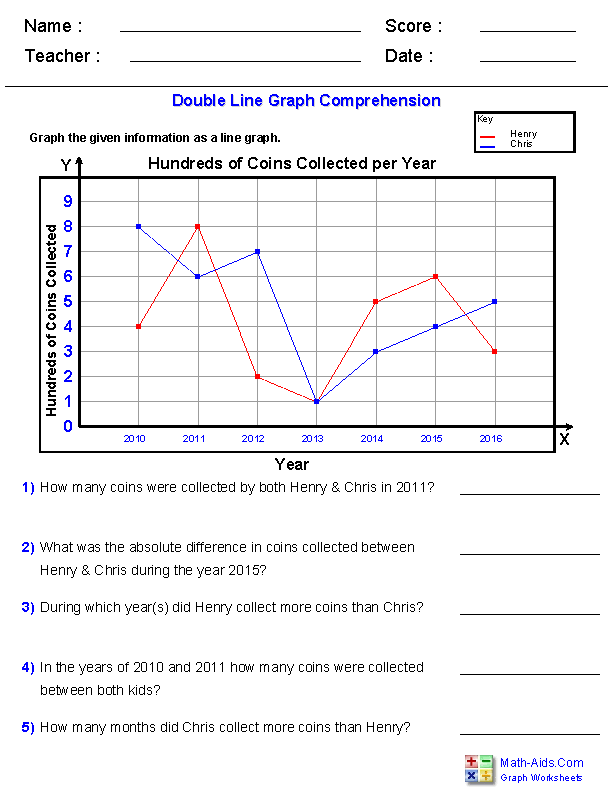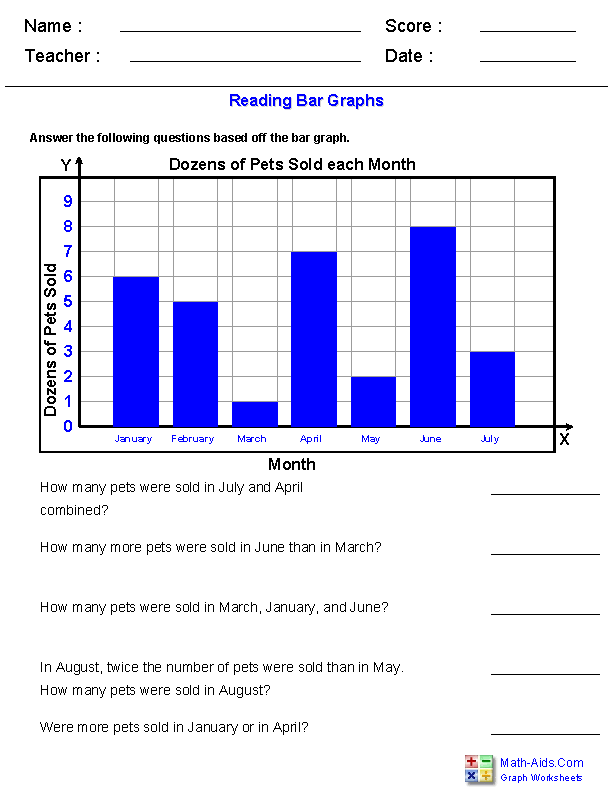##### Drawing Bar GraphsGraph Worksheets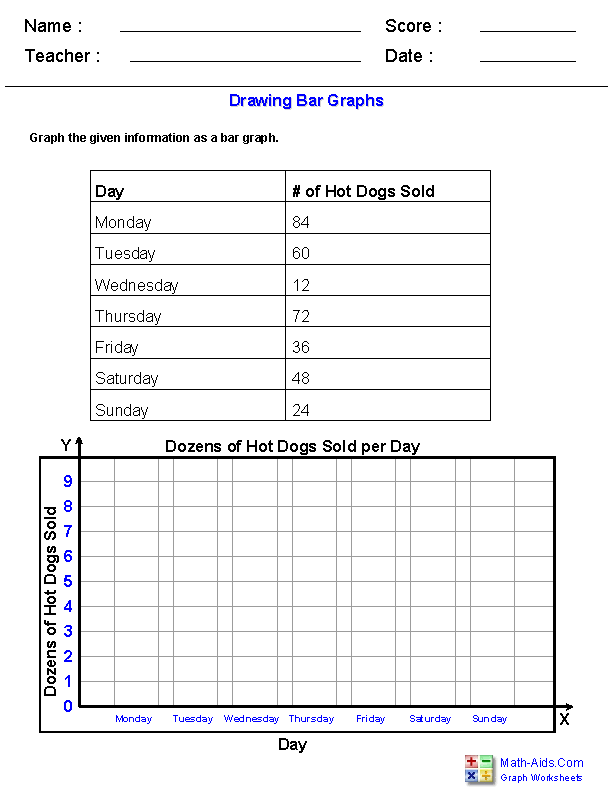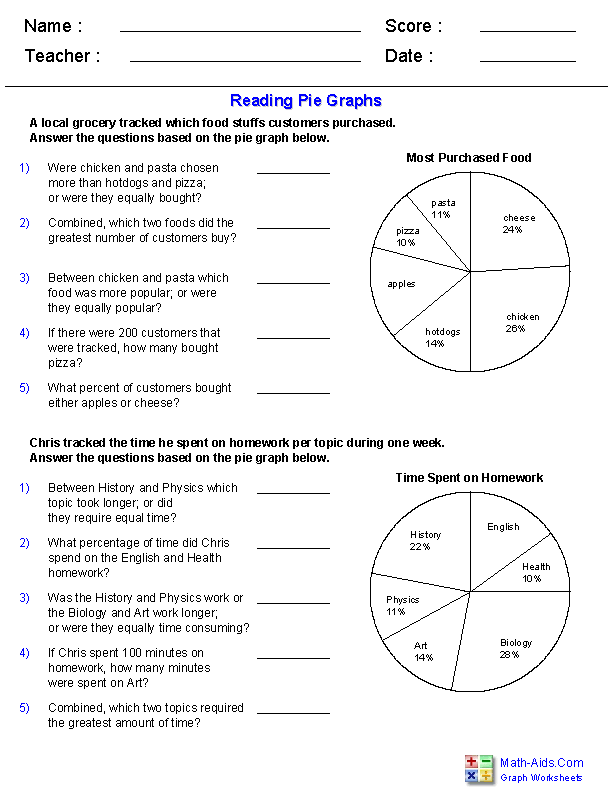##### Interpreting Line PlotsGraph Worksheets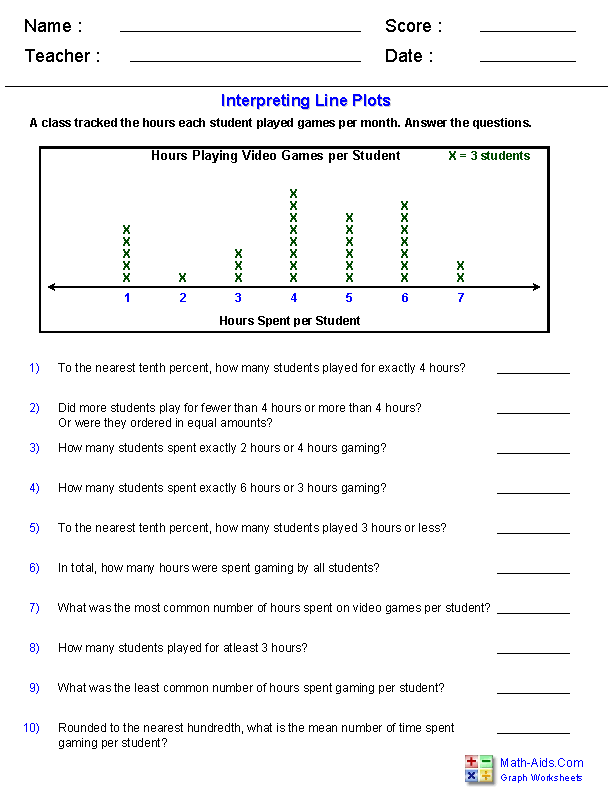##### Interpreting PictographsGraph Worksheets##### Box and Whisker PlotsGraph Worksheets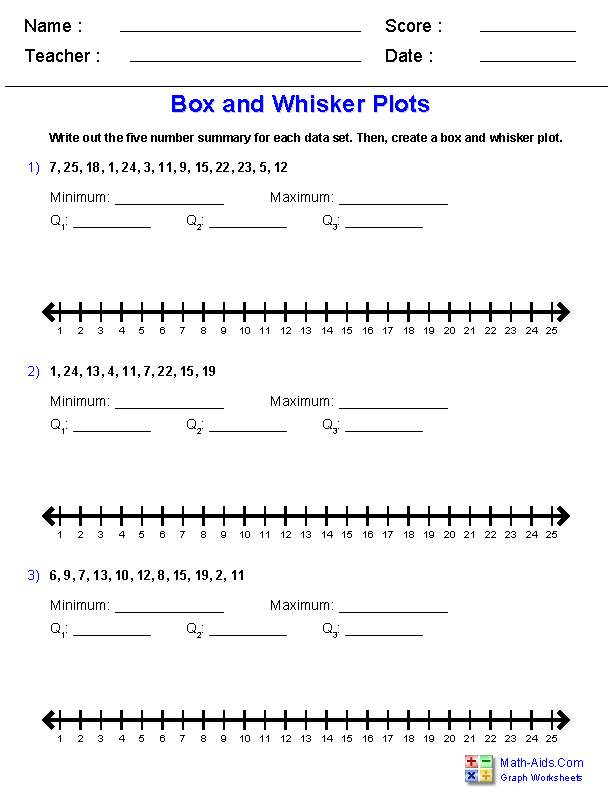##### Quartile-Five-Number-SummaryGraph Worksheets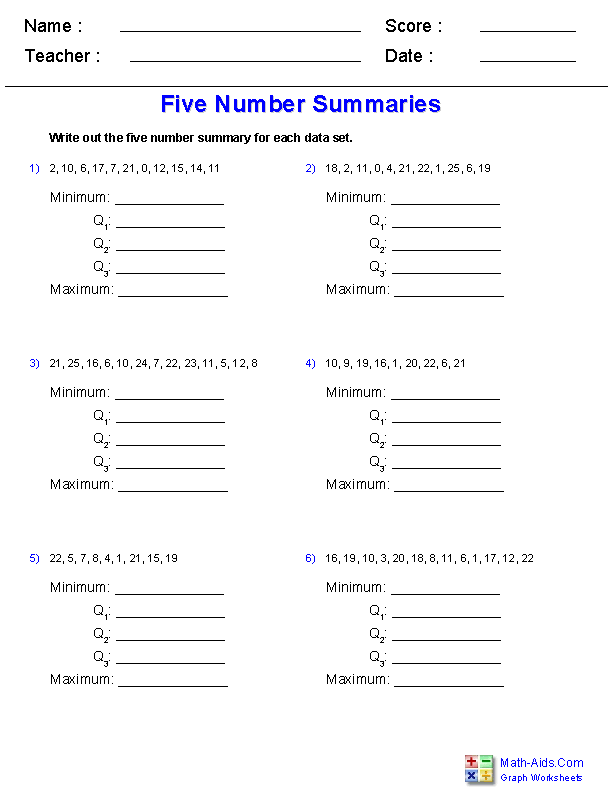##### Box and Whisker PlotsWord Problems WorksheetsRecommended Videos

## Detailed Description for All Graph Worksheets

Single Line Graphing Worksheets
These graph worksheets will produce a chart of data and a single coordinate grid to graph the data on. You may select the difficulty of the Graph task.

Single Line Graph Comprehensions Worksheets
These graph worksheets will produce a single coordinate line graph and questions based off the graph to answer. You may select the difficulty of the questions.

Double Line Graphing Worksheets
These graph worksheets will produce a chart of data for two lines and a single coordinate grid to graph the data on. You may select the difficulty of the graphing task.

Double Line Graph Comprehensions Worksheets
These graph worksheets will produce a single coordinate double line graph and questions based off the graph to answer. You may select the difficulty of the questions.

These graph worksheets will produce a bar graph, and questions to answer based off the graph. You may select the difficulty of the questions.

Drawing Bar Graphs Worksheets
These graph worksheets will produce a chart of data and a grid to draw the bar graph on. You may select the difficulty of the graphing task.

These graph worksheets will produce a pie graph, and questions to answer based off the graph. You may select the difficulty of the questions.

Interpreting Line Plots Worksheets
These graph worksheets will produce a line plot, and questions to answer based off the plot. You may select the difficulty of the questions.

Interpreting Pictographs Worksheets
These graph worksheets will produce a pictograph, and questions to answer based off the graph. You may select the difficulty of the questions.

Box and Whisker Plots Worksheets
These graph worksheets will produce a data set, of which the student will have to make a box and whisker plot. You may select the difficulty of the questions.

Quartile Five Number Summary Worksheets
These graph will produce a data set, of which the student will have to make a five number summary. You may select the amount of data, the range of numbers to use, as well as the number of problems. This worksheet is useful for teaching how to make box and whisker plots.

Box and Whisker Plots Word Problems Worksheets
These graph worksheets will produce data sets based off word problems, of which the student will have to make box and whisker plots. You may select the amount of data, the range of numbers to use, as well as how the data is sorted.